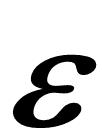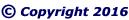"CLICK" below image; or, above blue ellipse to navigate.
 Emergent Ellipse Geometry Pythagorean Triangle Pairs (PTP)Construct a Pythagorean Triangle HIKin accordance with base, side, and hypotenuse as indicated in thebelow Table of Formulas. Pythagorean Triangles are triangleswith a right angle; and,all sides that are integers.
 x = Integer (base 10)=b/2
 Table of Formulas
 Pythagorean Triangle
 HIK = Pythagorean Vector Triangle
 HI = base (b), V-wave, (Vw) = 2x
 HK = V-radius (Vr)=b2/4 –= x2–IK = V-hypotenuse (Vh)=b2/4+= x2+The Pythagorean Theorem, a2 + b2 = c2, is an an incredible coincidence of number theory, which arises from the Emergent Ellipsoid and the Natural source of Mathematics.
 "CLICK" top image; or, below blue ellipse to navigate.One must continuously ask: Why? Why? Why?; and, Why? again. And, realize that Fundamental Nature is the source of all Mathematics!
 Summary Epsilon equals One Proof of One Inverse Square Law Elliptical Constant Duality of Infinity
 Natural Function Brunardot Theorem revised Fibonacci Sequence Challenge to Academe Pulsoid Theorem Fundamental Intrinsic Time
 Salient Structural Parts Universal Locus Antimatter Heaven/God/Hell Philogic Entanglement

--- Page 1 ---